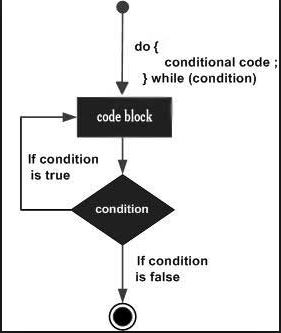# Do... While loops question

Tell us what’s happening:
The solution seen below works. What i don’t understand is why :
do{
myArray.push(i);
i++;
}while (i < 5) {
myArray.push(i);
i++;
}

would give me the result: myArray = [10, 11]. does ‘do’ mean that do{statement} will run, AND the while(condition){statement} will also iterate once despite the while’s condition??

``````
// Setup
var myArray = [];
var i = 10;

// Only change code below this line.
do{}
while (i < 5) {
myArray.push(i);
i++;
}

``````

User Agent is: `Mozilla/5.0 (Windows NT 10.0; Win64; x64) AppleWebKit/537.36 (KHTML, like Gecko) Chrome/67.0.3396.99 Safari/537.36`.

do while loops check the condition after the block is executed, i.e myArray.push(i),i++ (In your case).
do while loops is quite opposite to while loops.
while loop, which tests the condition before the code within the block is executed, the do-while loop is an exit-condition loop.

thank you, so the while portion of the loop runs despite the condition, and then runs the the do portion only once the while portion has been iterated once?? I take it that is what you mean by ‘exit-condition’

Exit-condition means that after executing the do statement , It checks the while statement whether it is correct or not, If it is incorrect then it will terminate the loop

then why wouldn’t myArray = ; and i = 11, after running the loop as expressed up top? Shouldn’t the while loop not run at all?
i is initiated to 10 btw.

Look the main condition in while is (i<5), Since you have initialized the i value to 10, Which is greater than 5, So the while statement is correct and calls the function myArray.push(i); and there is i++ also ,which increments the value by 1, i.e the value becomes 11. If u still face problem then look at the picture below and have a detail look on itthank you for being patient.
but you say ‘Look the main condition in while is (i<5), Since you have initialized the i value to 10, Which is greater than 5, So the while statement is correct’
But i is not less than 5. 10 < 5 is a false statement so the while loop shouldn’t execute, right?

i got it.
Sorry for the above thing…Yes. the while statement is not executed at all. Since the value of i is 10 , which is bigger than 5, so the while condition is false.And only the statements in the do loop are excecuted i.e MyArray.push(i){here i value is 10} and i++ ,i value will be increased…
I guess this is clear.

Hi,
The code below

…is the same as this just indented differently.

``````var myArray = [];
var i = 10;

// do while loop executes once no matter what
do {
myArray.push(i);
i++;
} while (i < 5);
console.log(myArray);  // 

// just some code in { } that executes
// not part of do...while loop
{
myArray.push(i);
i++;
}
console.log(myArray) // [10,11]
``````